#Function Repository Resource:

# BiPlot

Visualize the principal components of tabular data

Contributed by: Jon McLoone
 ResourceFunction["BiPlot"][data] generates a ListPlot of the first two principal components of data together with an arrow for each column to indicate the relationship with the principal components. ResourceFunction["BiPlot"][data, {a,b}] generates a ListPlot of the a and b principal components of data together with an arrow for each column to indicate the relationship with the principal components a and b. ResourceFunction["BiPlot"][data, {a,b,c}] generates a ListPointPlot3D of the a,b and c principal components of data together with a 3D arrow for each column to indicate the relationship with the principal components.

## Details and Options

Standard options for ListPlot and ListPointPlot3D are supported
 "ColumnNames" Names of data columns, used to label arrows in the plot “LabelPosition” Positioning of arrow labels as a proportion of arrow length "ArrowStyle" Graphics primatives to be applied to the arrows in the plot
BiPlot uses internally to compute principal components.

## Examples

### Basic Examples

In this data set we can see from the arrows that “Petal width” and “Petal length” are highly correlated, but “Sepel width” is largely independent from both:

 In:=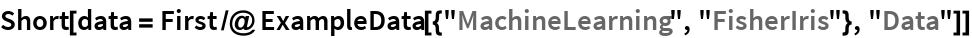Out=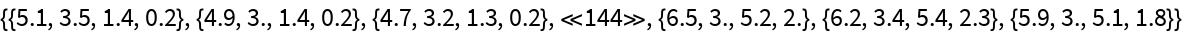In:=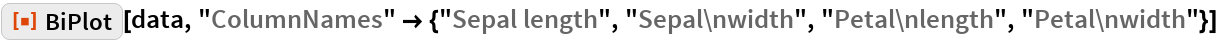Out=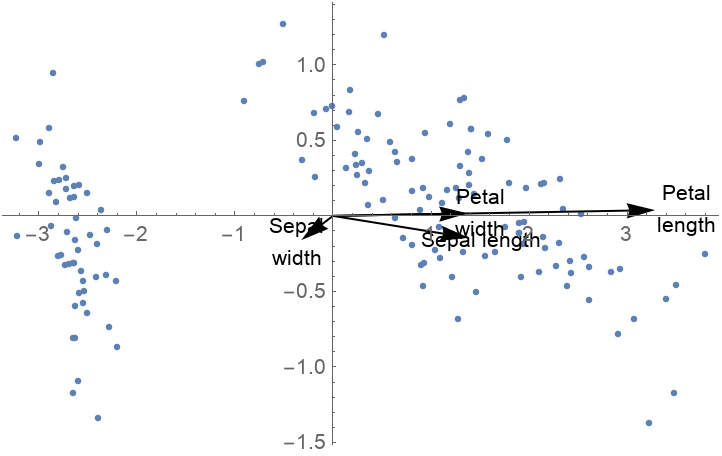### Scope

By default the first and second principal values are used, but you can specify different choices:

 In:=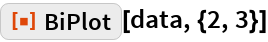Out=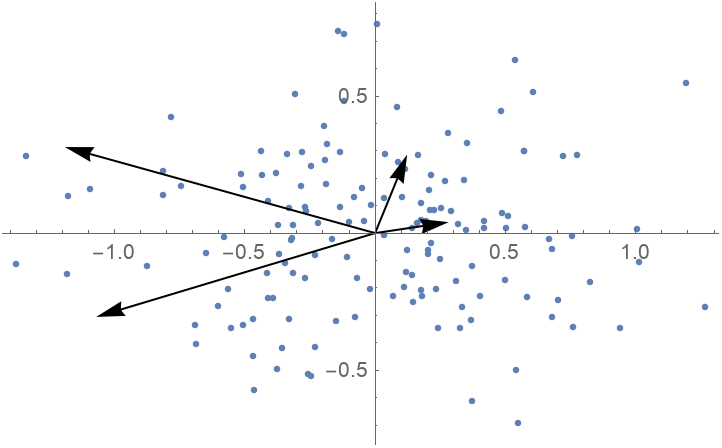If you specify three principal values, then the data and arrows are shown in three dimensions:

 In:=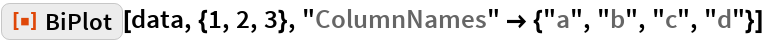Out=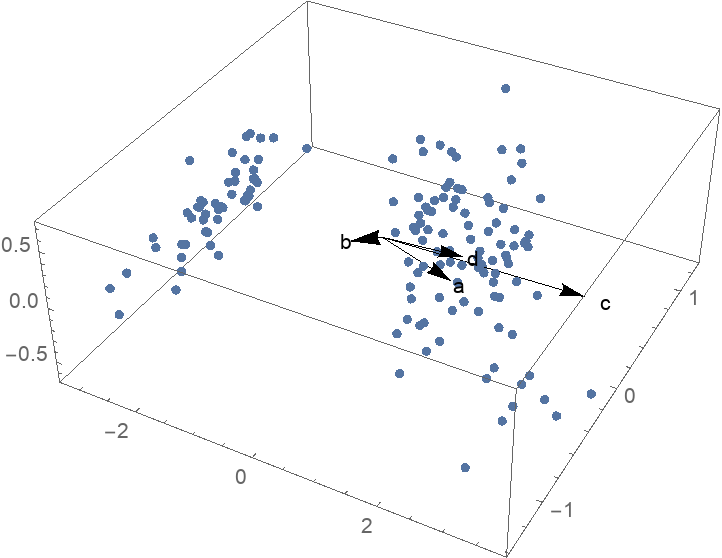If your data is a list of associations or a Dataset of a list of associations, then "ColumnNames" are chosen automatically and the arrows are labeled:

 In:=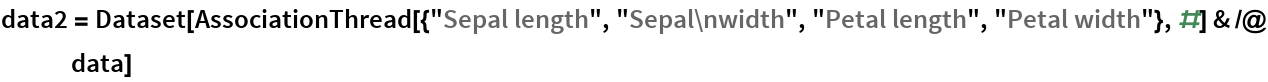Out=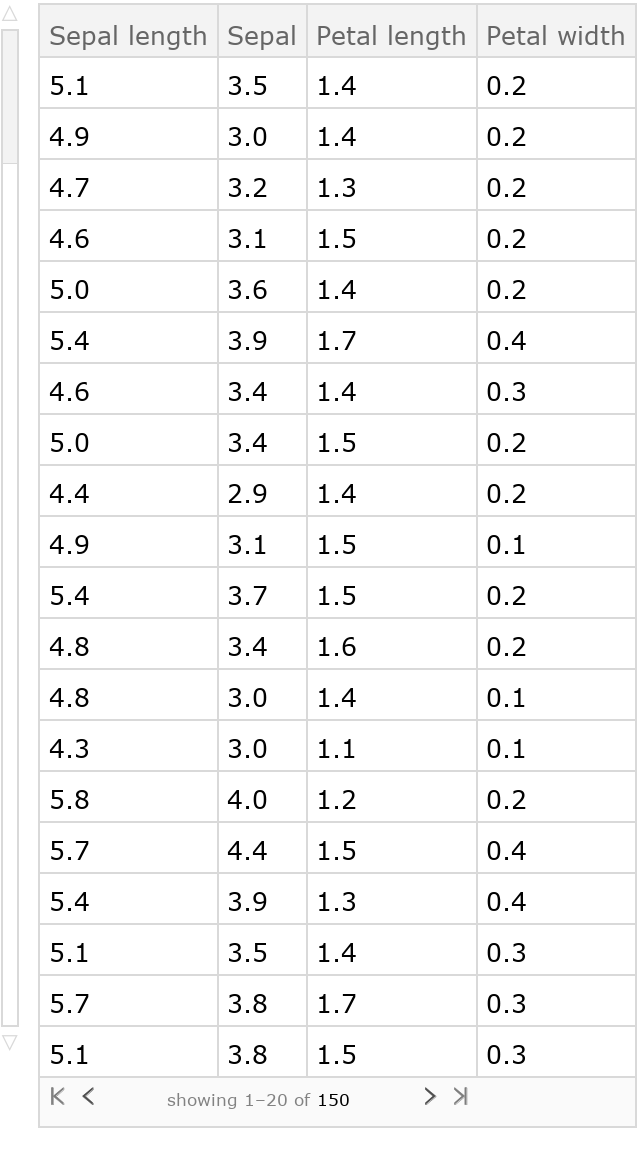In:=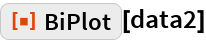Out=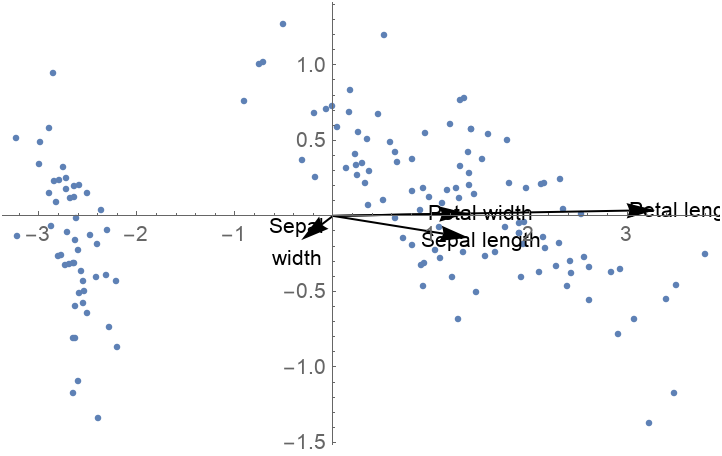### Options

"ColumnNames" can be used to place labels on the arrows:

 In:=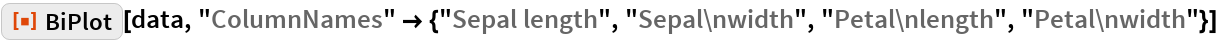Out=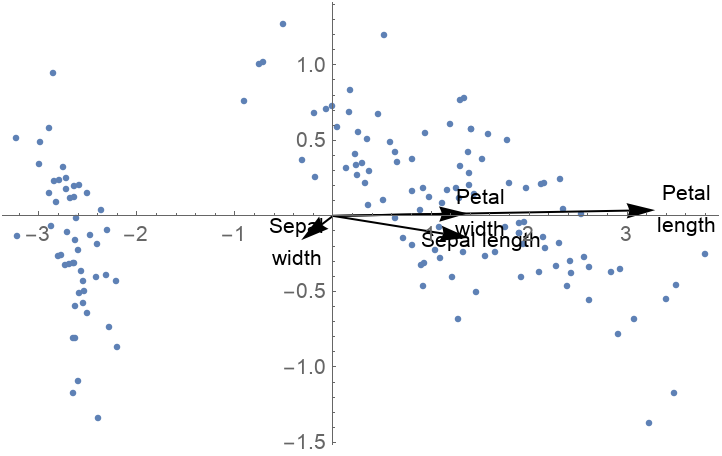"LabelPosition" can be used to position labels along or beyond the arrows:

 In:=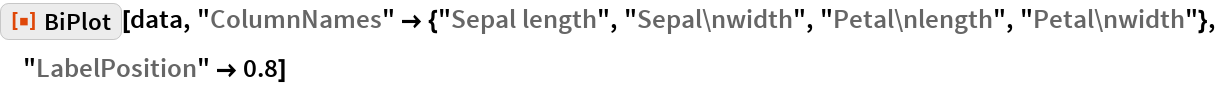Out=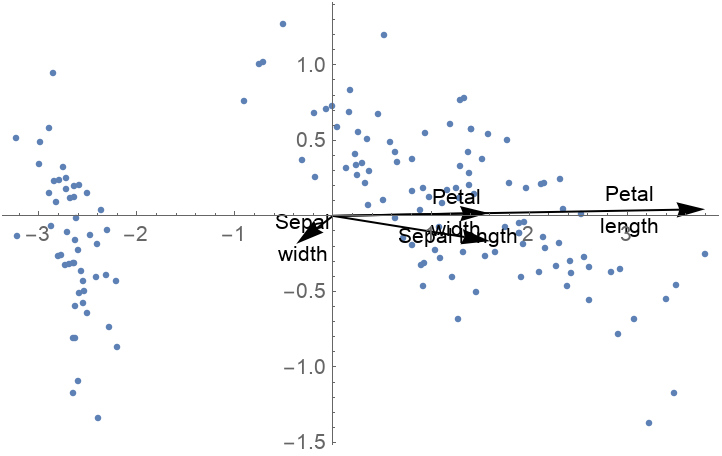"ArrowStyle" can be used to style the arrows:

 In:=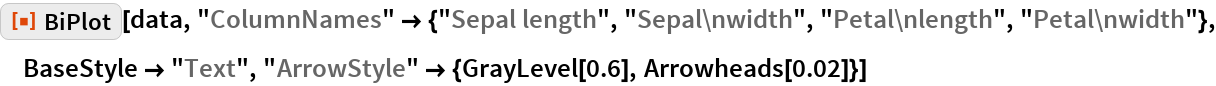Out=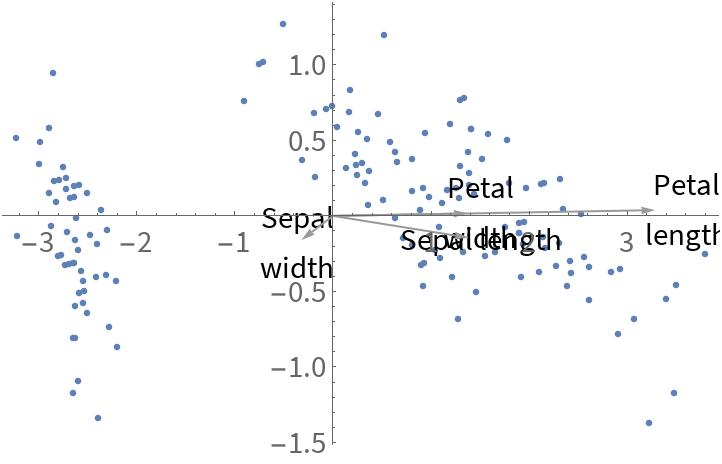## Requirements

Wolfram Language 11.3 (March 2018) or above